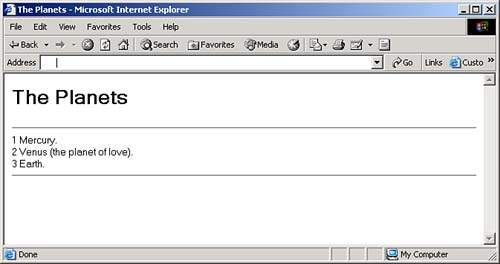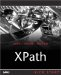# Using Context Functions

There is also a set of functions designed to get information about the evaluation context. Here are those functions, some of which we've seen in XPath 1.0:

• fn:position returns the position of the context item within the sequence of items currently being processed .

• fn:last returns the number of items in the sequence of items currently being processed.

• fn:current-dateTime returns the current xs:dateTime .

• fn: current-date returns the current xs:date .

• fn:current-time returns the current xs:time .

• fn: default-collation returns the value of the default collation property from the static context.

• fn: implicit-timezone returns the value of the implicit timezone property from the evaluation context.

We'll take a closer look at these functions here, starting with fn:position .

#### The fn:position Function

XPath 2.0 supports our old friend from XPath 1.0, the fn:position() function. In XPath 2.0, this function returns an xs:integer value holding the position of the context item among its siblings:

` `
` fn:position() as xs:integer? `

Here's an example; say that you wanted to format the names of the planets in our planetary data document like this (note the extra annotation for Venus):

` `
` 1 Mercury. 2 Venus (the planet of love). 3 Earth. `

To make this happen, we'll loop over all the <planet> elements using an <xsl:for-each> element:

` `
` <xsl:template match="planets">  <xsl:for-each select="planet">   .   .   .   </xsl:for-each>  </xsl:template> `

We can number each planet's name using the position function like this:

` `
` <xsl:template match="planets">    <xsl:for-each select="planet">  <xsl:value-of select="position()"/>   <xsl:text> </xsl:text>   <xsl:value-of select="name"/>   .   .   .  <br/>    </xsl:for-each> </xsl:template> `

And we can also add the annotation for Venus like this:

` `
` <xsl:template match="planets">    <xsl:for-each select="planet">        <xsl:value-of select="position()"/>        <xsl:text> </xsl:text>        <xsl:value-of select="name"/>  <xsl:value-of select="if (position() = 2) then   ' (the planet of love).' else '.'"/>   .   .   .  <br/>    </xsl:for-each> </xsl:template> `

All that's left is to separate each planet's entry onto a different line, and we'll use a <br/> element for that, as you can see in this example's code, ch09_06.xsl , which appears in Listing 9.6.

##### Listing 9.6 Using the fn:position Function ( ch09_06.xsl )
` <xsl:stylesheet version="2.0"     xmlns:xsl="http://www.w3.org/1999/XSL/Transform">     <xsl:template match="planets">        <xsl:for-each select="planet">            <xsl:value-of select="position()"/>            <xsl:text> </xsl:text>            <xsl:value-of select="name"/>            <xsl:value-of select="if (position() = 2) then                ' (the planet of love).' else '.'"/>  <br/>  <br/>        </xsl:for-each>     </xsl:template> </xsl:stylesheet> `

Here's what you get when you apply this stylesheet to our planetary data document:

` `
` C:\Saxon>java net.sf.saxon.Transform ch02_01.xml ch09_06.xsl <?xml version="1.0" encoding="UTF-8"?> 1 Mercury. <br/> 2 Venus (the planet of love). <br/> 3 Earth. <br/> `

#### The fn:last Function

The last() function in XPath 1.0 appears in XPath 2.0 as fn:last() , which returns an xs:integer indicating the number of items in the sequence of items currently being processed. Here's its signature:

` `
` fn:last() as xs:integer? `

THERE'S NO first() FUNCTION

As in XPath 1.0, there's no first() functioninstead, you test whether the position() function returns a value of 1.

Here's an example using the last() function. Say that we want to adapt our previous XSLT example to create an HTML document in which we enclose the planetary list with horizontal rule, <hr/> , elements. To convert the output of our XSLT stylesheet from XML to HTML, we only need to make the document element of the output document <html> , which causes the XSLT processor to assume the output document is HTML:

` `
` <xsl:template match="planets">  <html>   .   .   .   </html>  </xsl:template> `

Then we just add the other HTML we need, and add an <xsl:if> element to check for the beginning of the list, where we add the first <hr/> element:

` `
` <xsl:template match="planets">  <html>   <head>   <title>   The Planets   </title>   </head>   <body>   <h1>   The Planets   </h1>   <xsl:for-each select="planet">   <xsl:if test="position() = 1"><hr/></xsl:if>   <xsl:value-of select="position()"/>   <xsl:text> </xsl:text>   <xsl:value-of select="name"/>   <xsl:value-of select="if (position() = 2)   then ' (the planet of love).'   else '.'"/>   .   .   .   <br/>   </xsl:for-each>   </body>  </html> </xsl:template> `

All that's left is to add the final <hr/> element at the end of the list, and that works as you can see in ch09_07.xsl (Listing 9.7).

##### Listing 9.7 Using the fn:last Function ( ch09_07.xsl )
` <xsl:stylesheet version="2.0"     xmlns:xsl="http://www.w3.org/1999/XSL/Transform">     <xsl:template match="planets">         <html>             <head>                 <title>                     The Planets                 </title>             </head>             <body>                 <h1>                     The Planets                 </h1>                 <xsl:for-each select="planet">                     <xsl:if test="position() = 1"><hr/></xsl:if>                     <xsl:value-of select="position()"/>                     <xsl:text> </xsl:text>                     <xsl:value-of select="name"/>                     <xsl:value-of select="if (position() = 2)                         then ' (the planet of love).'                         else '.'"/>  <xsl:if test="position() = last()"><hr/></xsl:if>  <br/>                 </xsl:for-each>             </body>         </html>     </xsl:template> </xsl:stylesheet> `

To store our results in an HTML document named results.html , you can use this command with Saxon:

` `
` C:\Saxon>java net.sf.saxon.Transform ch02_01.xml ch09_07.xsl > results.html `

And you can see the result in Figure 9.1.

##### Figure 9.1. Using the last() function.#### The fn:current-dateTime Function

This function returns the current date and time, with timezone. Here's the signature of this function:

` `
` fn:current-dateTime() as dateTime `

This function returns an xs:dateTime value, and you can see an example in ch09_08.xsl , Listing 9.8, where we're just displaying the current time and date.

##### Listing 9.8 Using the fn:current-dateTime Function ( ch09_08.xsl )
` <xsl:stylesheet version="2.0"     xmlns:xsl="http://www.w3.org/1999/XSL/Transform">     <xsl:template match="/">  <xsl:value-of select="current-dateTime()"/>  </xsl:template> </xsl:stylesheet> `

Here's the kind of result you get when you run this example through Saxon:

` `
` <?xml version="1.0" encoding="UTF-8"?> 2003-09-19T17:00:25.255Z `

#### The fn:current-date Function

The fn:current-date() function just returns an xs:date object, with timezone information, that holds the current date. Here's how you use this function:

` `
` fn:current-date() as xs:date `

You can see an example in ch09_09.xsl , Listing 9.9, where we're displaying the current date.

##### Listing 9.9 Using the fn:current-date Function ( ch09_09.xsl )
` <xsl:stylesheet version="2.0"     xmlns:xsl="http://www.w3.org/1999/XSL/Transform">     <xsl:template match="/">  <xsl:value-of select="current-date()"/>  </xsl:template> </xsl:stylesheet> `

Here's the kind of result you get from Saxon using this example:

` `
` <?xml version="1.0" encoding="UTF-8"?> 2003-09-19 `

#### The fn:current-time Function

As you can gather from its name, the fn:current-time() function returns the current time. Here's how you use it:

` `
` fn:current-time() as time `

The return value is an xs:time value, including timezone. You can see an example in ch09_10.xsl (Listing 9.10).

##### Listing 9.10 Using the fn:current-time Function ( ch09_10.xsl )
` <xsl:stylesheet version="2.0"     xmlns:xsl="http://www.w3.org/1999/XSL/Transform">     <xsl:template match="/">  <xsl:value-of select="current-time()"/>  </xsl:template> </xsl:stylesheet> `

And here's what you might see when you run this example:

` `
` <?xml version="1.0" encoding="UTF-8"?> 17:24:23.503Z `XPath Kick Start: Navigating XML with XPath 1.0 and 2.0
ISBN: 0672324113
EAN: 2147483647
Year: 2002
Pages: 131

Similar book on Amazon